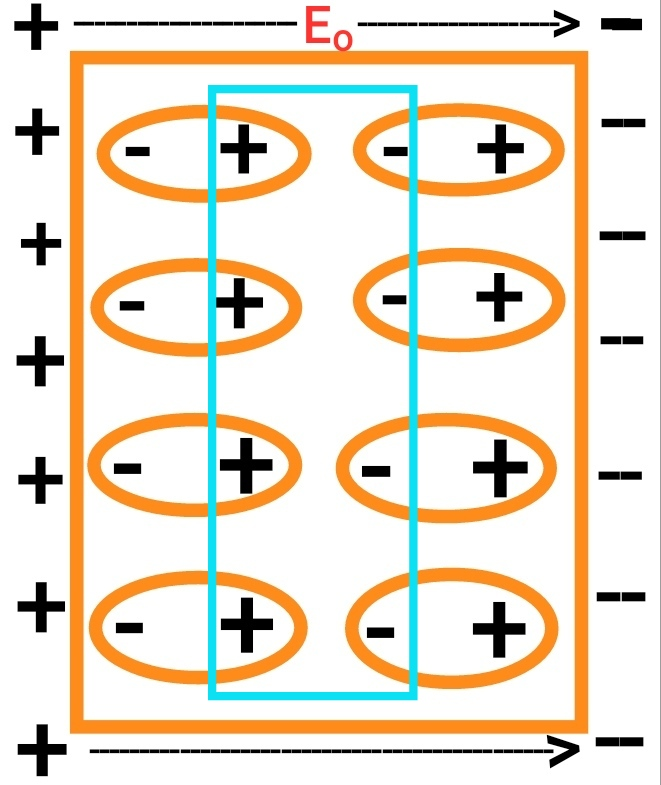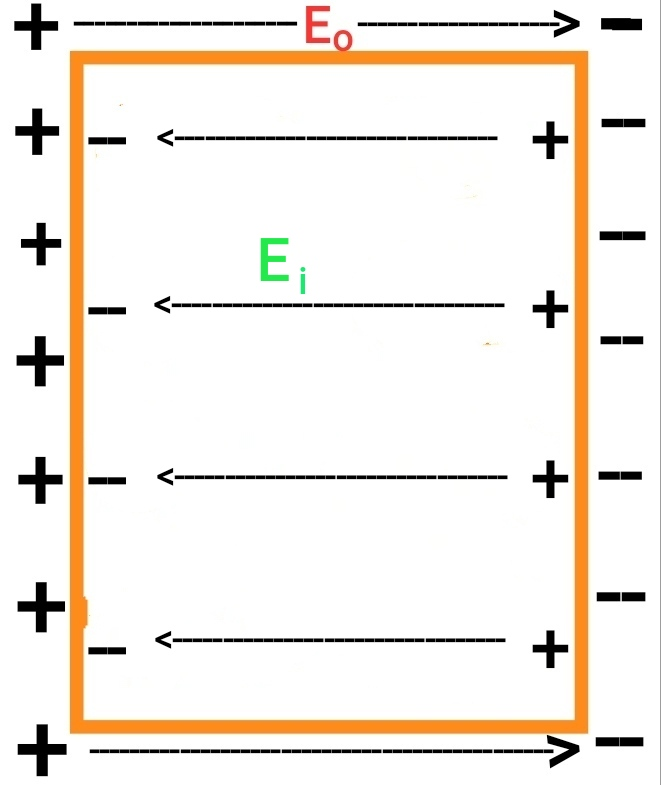# Electric Polarization & Dielectric Constant

Electric Polarization:

Electric polarization is defined as  the dipole moment induced per unit volume of a dielectric, when the dielectric is placed in an external electric field.

consider a non-polar dielectric slab , placed in an external electric field so that each of the atom is polarized along the applied electric field. We consider that the distance between centre of positive charges and centre of negative charges in the atom is x. Hence the dipole moment of each atom is

p  =  qx

is N is the number of atoms per unit volume then dipole moment per unit volume is

P = N p

Or.   P  = N q x

The electric polarization is also called is dipole moment density and its unit is coulomb per metre square ( C m-2 ).

As an external field is applied in the dielectric, charges are induced in the surface of the dielectric, perpendicular to the direction of electric field as shown in figure.Or.Hence, the dielectric produces only surface charge density. Due to these induced charges or polarization charges an electric field Ei is set up inside the dielectric in a direction opposite to the direction of applied electric field Eo and is called induced electric field (Ei).

Hence the effective value or reduced value of electric field in a polarized dielectric is

E = Eo - Ei

The value of E depends upon the nature of the dielectric slab.

Dielectric constant:

The ratio of the external electric field Eo to the reduced value of electric field E is found to be always a constant for a given material. This constant is known as dielectric constant of the material of the dielectric.

Dielectric constant is generally denoted by K and is given by

K = Eo / E

Since the dielectric always decreases the applied electric field , that is E < Eo,

Hence, K > 1